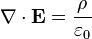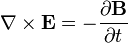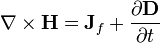## Partial differential equations

A Partial differential equation is a differential equation that contains unknown multivariable functions and their partial derivatives. They are used to formulate problems involving functions of several variables. They can either be solved by hand or used to create a relevant computer módel.

Almost all the elementary and numerous advanced parts of theoretical physics are formulated in terms of differential equations. Often partial differential equations.

The most frequently encountered are:

1. Laplace’s equation: 2   ψ = 0. Important in the study of electromagnetic phenomena, dielectrics, steady currents and magnetostatics, hydrodynamics (irrotational flow of a perfect fluid, and surface waves, heat flow, gravitation.)
2. Poisson’s equation:∇2   ψ = ρ/ε. Non-homogenous with a source term.
3. The Helmholtz equation or wave equation:

2   ψ+ k2ψ = 0 and time-independent diffusion equations. This equation can be used to describe : elastic waves in solids, bars, membranes, sound, acoustic waves, nuclear reactors

1. The time dependent diffusion equation: ψ= δψ/δta-2 .
2. The time-dependent wave-equation □2ψ =0.
3. The scalar potential equation. □2ψ = -ρ/ε.
4. The Klein-Gordon equation □2ψ =μψ
5. The Schrödinger equation

-h2/(2m)∇ψ + V ψ = Eψ describing the motion of the sub-atomic particles.

1. Maxwell’s coupled differential equationsfor electric and magnetic field and Dirac’s equation for the relativistic electron wave
2. function.
3. Differential form
Name ”Microscopic” equations ”Macroscopic” equations
Gauss’s lawGauss’s law for magnetism